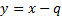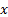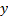/

### Constrained Quadratic Optimisation: 5. Finishing the algorithm

Next page

5.            Finishing the algorithm

The algorithm stops when there is no longer any valid combination of entering and leaving basic variables that improves the objective function. If the corresponding solution to the original problem is still not feasible (i.e. there are still some of the additional artificial variables introduced when setting up the super-problem that are greater than zero) then the original problem didn’t have a feasible solution. Otherwise, the solution to the original problem is the same as that for the super-problem. If we introduced a change of variablesat the start of the problem because the lower limits onwere not 0 then we need to unwind this change of variables, as the super-problem solution is defined in terms ofnot.